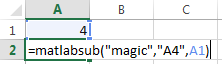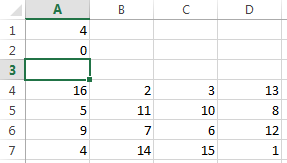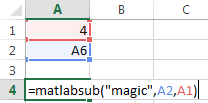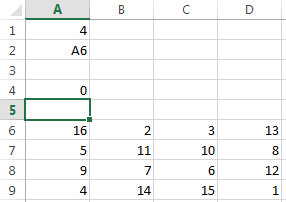matlabsub

Evaluate MATLAB command given Microsoft Excel data and designate output location

Description

example

= matlabsub(command,result,inputs) specifies the MATLAB® command for evaluation in the MATLAB workspace, given the input data inputs. The function returns the MATLAB output into the worksheet cell specified by result. Use this syntax when working in a worksheet cell.

Examples

collapse all

Enter the number four in cell A1.

Enter this text into cell A2. Specify the function magic as the command. Return the fourth-order magic square into the range of cells starting in cell A4. Reference cell A1 as the input argument for the magic function.

=matlabsub("magic","A4",A1)The fourth-order magic square displays in the range of cells from A4 through D7.Enter the number four in cell A1. Enter a target cell reference to cell A6 in cell A2.

Enter this text into cell A4. Specify the function magic as the command. Return the fourth-order magic square into the range of cells starting in cell A6 by entering the cell reference A2. Reference cell A1 as the input argument for the magic function.

=matlabsub("magic",A2,A1)The fourth-order magic square displays in the range of cells from A6 through D9.Input Arguments

collapse all

MATLAB command to evaluate, specified as a string. Enclose the string in double quotes. Or, enter the string in a cell without quotes and enter the corresponding cell reference without quotes as the input argument.

Example: "sum"

Example: A1

MATLAB result, specified as an Excel cell reference or range name that designates the location where to display the result of the MATLAB command. To return the result in a specific cell, specify the cell reference enclosed in quotes. To denote a worksheet cell address or range name that contains a cell reference to another cell, specify the cell reference without quotes.

Example: "A3"

Example: D6

MATLAB command input arguments, specified as an Excel cell reference or a reference to a range of cells. To specify multiple input arguments for a function, separate the cell references with commas.

Example: B1:B10

Example: A1:C1,A3

Tips

• To return an array of data to the Microsoft® Excel Visual Basic® for Applications (VBA) workspace, see MLEvalString and MLGetVar.

• result must not include the cell that contains matlabsub. Do not overwrite the function itself.

• Ensure that there is enough room in the worksheet for writing matrix contents. If there is insufficient room, the function generates a fatal error.

• If matlabsub fails, a standard Spreadsheet Link™ error displays by default; for example, #COMMAND. To return MATLAB errors, use MLShowMatlabErrors.

Introduced before R2006a